# Loss Function

This is the key component of prediction games considered in competitive on-line prediction. Formally, a loss function is any function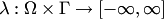, where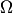is the outcome space and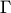is the prediction space. It measures the discrepancy between the prediction and the actual outcome. All algorithms for competitive on-line prediction make some assumptions about the loss function. Among the most popular classes of loss functions are: nonnegative, bounded, perfectly mixable, continuous in the second argument, having a bounded derivative in the second argument. The most popular loss functions in the case where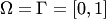are:

• The square loss function, sometimes also called the Brier loss function: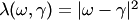.
• The absolute loss function: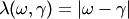.
• The Hellinger loss function: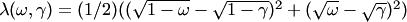.
• The log loss, or logarithmic loss, or relative entropy loss function: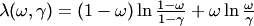. This loss function is unbounded.

The counting loss function used in the simple prediction game is the restriction of the absolute loss function (or the square loss function, or the Hellinger loss function) to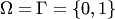.

Some algorithms consider the expected loss rather than the "pure" loss.

Some algorithms are capable of competing under discounted loss, where at each stepthe loss on all the previous steps is discounted with a factor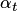. One of such algorithms is the Shortcut Defensive Forecasting, as it is shown here.

The important subclass of loss functions are proper scoring rules. The square loss and the logarithmic loss are proper.

One can easily generalize some of the losses given above to the case of multi-dimensional outcomes.